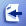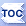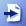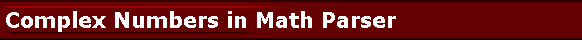# Using complex numbers with Math Parser

Some scientific applications require the use of complex numbers . The user will input an expression that contains complex numbers and this user supplied formula will need to be parsed and evaluated by the mathematical expression parser in your software. Bestcode Math Parser for C++ comes with support and usage examples for the complex C++ type in the standard C++ template library (STL). You can plug in the std::complex type to your parser type declaration and set the preprocessor directive _COMPLEX for it all to work.

 #include #include //If you are going to use the parser with std::complex, then define this. #define _COMPLEX #include "MathParser.h" using namespace std; //Define a Math Parser that works with char strings for //variable and function names, and complex values. typedef CMathParser > ComplexParser; int main(char **args){         cout << "Math Parser Example Expressions" << endl;         try{                 ComplexParser cp;                 cp.SetExpression("X^2+1+i"); //Variable i=sqrt(-1) is predefined by the parser.                 //cp.SetExpression("sqrt(-1)"); //result is i.                 cp.SetX(complex(0,1)); //value i is represented as (0,1).                         cout << "Complex expression:" << cp.GetExpression() << endl;                 cout << "Complex result    :" << cp.GetValue() << endl;         }catch(ComplexParser::ParserException &ex ){                 cout << ex.GetMessage() << endl;                 cout << "Invalid portion of expression is <" << ex.GetInvalidPortionOfExpression() << ">." << endl;         }catch(...){                 cout << "Unexpected error in math parser." << endl;         }         char c;         cin >> c; }

When executed, the output of this example is:

Math Parser Example Expressions
Complex expression:X^2+1+i
Complex result   :(0,1)

webmaster@gobestcode.com